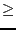Next: Cassini ovals Up: Gerschgorin circles Previous: Mathematical background   Contents

Implementation

It is possible to get these bounds by using the procedure:

int Gerschgorin(INTERVAL_MATRIX &A,int Size, int Type, INTERVAL &Bound)
where:
• A: the matrix
• Size: the dimension of the matrix
• Type: 0 if the matrix is not available, 2 if the matrix is symmetrical, 1 otherwise
• Bound: all the roots will be enclosed in this interval
the procedure returns 1 if it has been possible to compute Bound.

A more complete procedure allows to get all the Gerschgorin circles and eventually to adjust an interval that is supposed to contain the roots of the polynomial:

int Gerschgorin_Simplification(INTERVAL_MATRIX &A,int Size,int Type,
INTERVAL &Input,INTERVAL_VECTOR &Circle)
The arguments are the same than the previous procedure except for Input which is the interval supposed to contain roots of the polynomial and Circle which contain the projection of the Gerschgorin circle on the real axis. This procedure returns:
• -1: Input does not contain a root of the polynomial
• 0: no change in Input
•1: Input has been improved and the return value gives the number of distinct circlesNext: Cassini ovals Up: Gerschgorin circles Previous: Mathematical background   Contents
Jean-Pierre Merlet 2012-12-20